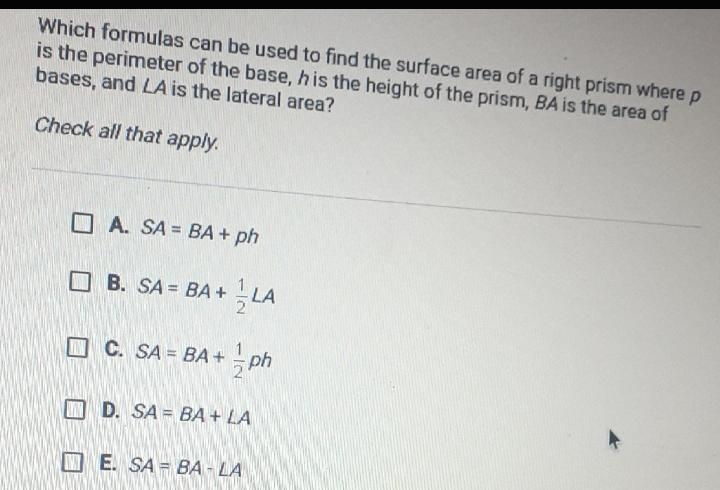Question:

# Which formulas can be used to find the surface area of a right prism where p is the perimeter of the base, h is the height of the prism, BA is the area of bases, and LA is the lateral area? Check allWhich formulas can be used to find the surface area of a right prism where p is the perimeter of the base, h is the height of the prism, BA is the area of bases, and LA is the lateral area? Check all that apply. A. SA = BA + ph B. SA = BA+ 1/2 LA C. SA = BA + 1/2 ph D. SA = BA + LA E. SA - BA - LA